# Excel Course: Conditional Formatting (examples 4 to 6)

## 4. Identify People Whose Names Are Missing from a Table

To determine whether a person whose name appears on a list is missing from a table, we will use the COUNTIF function.

The COUNTIF function will count the number of times that a first name appears in the table. If the result is 0, the formatting is applied.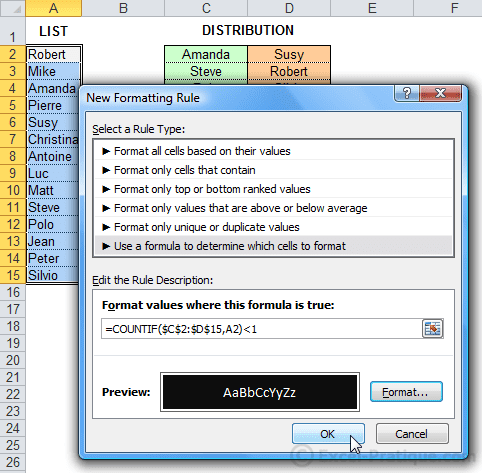Missing first names have been highlighted.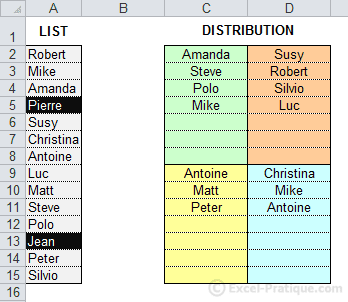You can use the same formula to find duplicate first names merely by replacing `<1` with `>1`.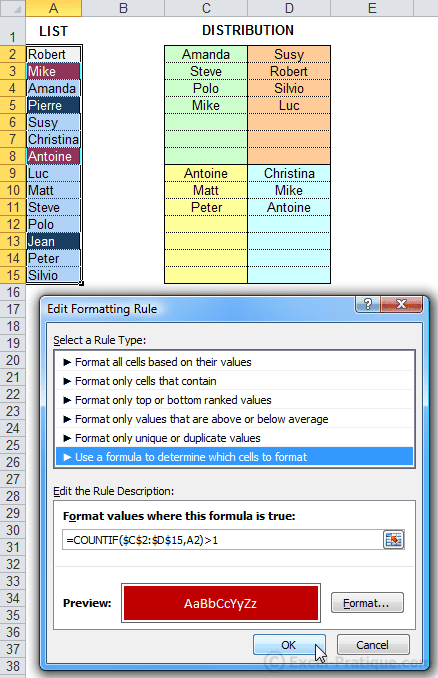Source file: cf4.xlsx

## 5. Shade Every Other Row

We will use the MOD function to shade every other row.

This function returns the remainder of division (in this case, division by 2). An even number will have a remainder of 0, but an odd number will have a remainder of 1.The CF uses the row number for the calculation.For checkerboard-style formatting, add `+COLUMN()`.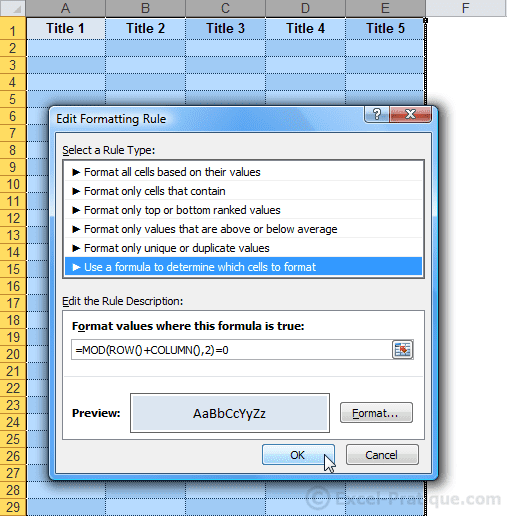Source file: cf5.xlsx

## 6. Search for a Word in the Text and Shade the Whole Row

The formula used here is `=SEARCH(\$B\$9;\$D2;1)`.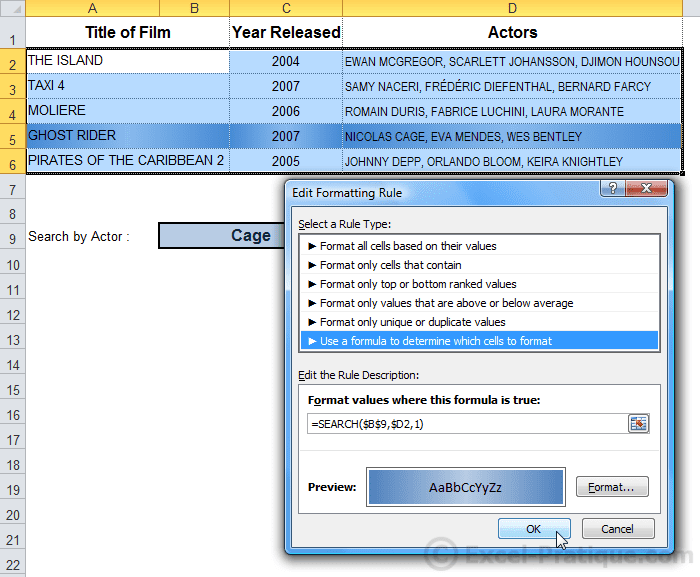If the word entered in B9 is found in any of the cells in Column D, the whole row will be shaded.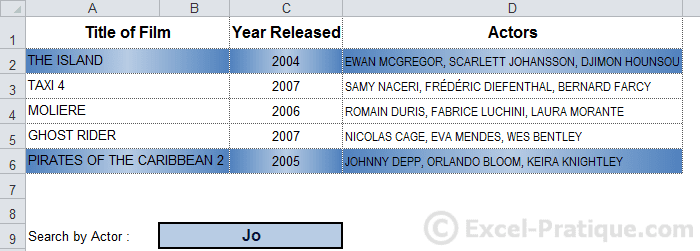Source file: cf6.xlsx